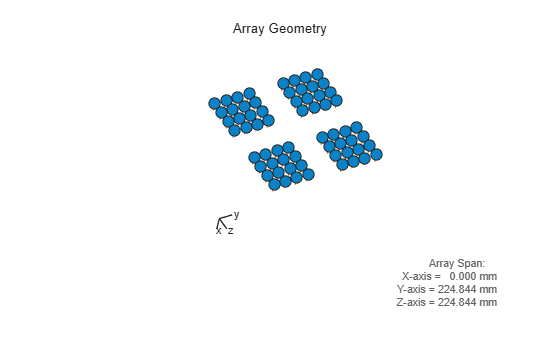# viewArray

View array geometry

## Syntax

``viewArray(array)``
``viewArray(array,Name,Value)``
``hndl = viewArray(___)``

## Description

````viewArray(array)` displays the geometry of the `array`.```

example

````viewArray(array,Name,Value)` displays the geometry of the array, with additional options specified by one or more `Name,Value` pair arguments.```
````hndl = viewArray(___)` returns the handle of the array elements in the figure window. All input arguments described for the previous syntaxes also apply here.```

## Examples

collapse all

Construct a 5G antenna array where the grid is 2-by-2 and each panel is 4-by-4 array. Each antenna element consists of two short-dipole antennas with different dipole axis directions. The antenna elements are spaced 1/2 wavelength apart and the panels are spaced 3 wavelengths apart. The array operates at 6 GHz.

```c = physconst('LightSpeed'); fc = 6.0e9; lambda = c/fc; antenna1 = phased.ShortDipoleAntennaElement('AxisDirection','Z'); antenna2 = phased.ShortDipoleAntennaElement('AxisDirection','X'); array = phased.NRRectangularPanelArray('ElementSet', ... {antenna1, antenna2},'Size',[4, 4, 2, 2], ... 'Spacing',[0.5*lambda,0.5*lambda,3*lambda,3*lambda]); viewArray(array,'ShowNormals',true, ... 'ShowLocalCoordinates',true,'Orientation',[60;100;45], ... 'ShowAnnotation',true)```## Input Arguments

collapse all

Phased array, specified as a System object.

### Name-Value Arguments

Specify optional comma-separated pairs of `Name,Value` arguments. `Name` is the argument name and `Value` is the corresponding value. `Name` must appear inside quotes. You can specify several name and value pair arguments in any order as `Name1,Value1,...,NameN,ValueN`.

Example: `'ShowNormals',true,'ShowIndex','All','ShowTaper',true`

Handle to the axes along which the array geometry is displayed.

Option to show normal directions, specified as the comma-separated pair consisting of `'ShowNormals'` and a Boolean value.

• `true` — show the normal directions of all elements in the array

• `false` — plot the elements without showing normal directions

Example: `false`

Data Types: `logical`

Logical flag specifying whether to show the local coordinate axes.

Data Types: `logical`

Logical flag specifying whether to show the annotations in the UI panel of the figure. Annotation shows aperture size and element spacing based on array axis of array.

Data Types: `logical`

Orientation of the array, specified as a 3-by-1 column vector containing the rotation angles with respect to the x-, y-, and z-axes of the local coordinate system, respectively. The default value is [0;0;0].

Data Types: `double`

Option to show taper magnitude, specified as the comma-separated pair consisting of `'ShowTaper'` and a Boolean value.

• `true` — change the element color brightness in proportion to the element taper magnitude

• `false` — plot all elements using the same color

Example: `true`

Data Types: `logical`

Element indices to show in the figure, specified as the comma-separated pair consisting of `'ShowIndex'` and a vector of positive integers. Each number in the vector must be an integer between 1 and the number of elements. To show all of indices of the array, specify `'All'`. To suppress all indices, specify `'None'`.

Example: `[1,2,3]`

Data Types: `double`

Plot title, specified as a character vector.

Example: `'My array plot'`

Data Types: `char` | `string`

## Output Arguments

collapse all

Handle of array elements plot in the figure window, returned as a scalar.

Introduced in R2021a